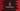# C program to find the nth Armstrong number## C program to find the nth Armstrong number:

In this post, we will learn how to find the nth Armstrong number in C. The program will take a number as input from the user and it will print the nth Armstrong number.

We will also learn how to find if a number is an Armstrong number or not.

### Algorithm:

Before we move to the Algorithm, let’s understand what is an Armstrong number. A number of digit n is called an Armstrong number if the sum of each digit of the number raised to n is equal to the number itself.

For example, 153 is an Armstrong number. Because,

• The total digit of 153 is 3
• The sum of power of each digit to 3 is:
``1^3 + 5^3 + 3^3 = 1 + 125 + 27 = 153``

i.e. the sum is equal to the number. So, it is an Armstrong number.

To find the nth Armstrong number, we will run a loop starting from 1. For each digit of the loop, it will check if it is an Armstrong number or not. If yes, it will print the number.

We can also take the lower limit to use in the loop. For example, we can start the loop from 100 and it will find the nth Armstrong number starting from 100.

### C program:

Let’s write down the program in C:

``````#include <stdio.h>

int getTotalDigits(int n)
{
int total = 0;
while (n > 0)
{
n /= 10;
total++;
}
}

int power(int n, int p)
{
int power = 1;
while (p > 0)
{
power *= n;
p--;
}
return power;
}

int isArmstrong(int n)
{
int digits = getTotalDigits(n);
int numCopy = n;
int sum = 0;
int lastDigit;

while (numCopy > 0)
{
lastDigit = (int)(numCopy % 10);
sum += power(lastDigit, digits);
numCopy = numCopy / 10;
}
return n == sum;
}

int main()
{
int n;
int armstrong;
printf("Enter the value of n: ");

scanf("%d", &n);

int i = 1;

while (n > 0)
{
if (isArmstrong(i))
{
armstrong = i;
n--;
}
i++;
}

printf("Armstrong number: %d\n", armstrong);
}``````

Here,

• getTotalDigits method is used to get total number of digits in a number.
• power method takes two parameter and returns the power of n^p
• isArmStrong method takes a number as its parameter and checks if it is an Armstrong number or not. It returns 1 if it is an Armstrong number. Else, it returns 0.
• The program takes the value of n as an input from the user and runs one while loop from 1.
• The while loop stops once it finds the nth Armstrong number.
• The last line is printing the value of nth Armstrong number.

### Sample Output:

The first 17 Armstrong numbers are: 1, 2, 3, 4, 5, 6, 7, 8, 9, 153, 370, 371, 407, 1634, 8208, 9474, 54748. Now, let’s use the above program to find the nth Armstrong value:

``````Enter the value of n: 10
Armstrong number: 153

Enter the value of n: 17
Armstrong number: 54748``````

As you can see here, it returns the nth Armstrong number.